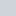## Cymath Math Problem Solver 2.17 APK(No Ratings Yet)Loading...With millions of users worldwide Cymath.com, the Cymath math problem-solving app uses the same math engine while allowing you to solve problems on your travels! Just enter a problem with your math homework, and let Cymath solve for you step by step! We provide algebra, as well as calculus help.
Subjects in algebra include: solving the equation, factorization, logarithms, exponents, complex numbers, second degree equations, trigonometry, partial fraction, polynomial division, and so on. Topics in the calculation include: product rule, quotient rule, string rule, u-substitution, part insertion, partial fraction integration, trigonometric substitution, rationalization substitution, and more again. We provide mathematical answers and steps for all of the above.

Supported Android
{4.1 and UP}
Supported Android Version:-
Jelly Bean (4.1–4.3.1) - KitKat (4.4–4.4.4) - Lollipop (5.0–5.0.2) - Marshmallow (6.0 - 6.0.1) - Nougat (7.0 – 7.1.1) - Oreo (8.0-8.1)

# Download Cymath Math Problem Solver 2.17 APK For Android

Cymath Math Problem Solver Plus v2.16.apk